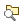## Dependency Analyzer Scope and Limitations

### Analysis Scope

The Dependency Analyzer identifies the required files and toolboxes for your project or model. The analysis covers a wide range of dependencies, including model references, subsystem references, linked libraries, MATLAB® and `C/C++` code, Stateflow® charts, data files, S-functions, and requirements documents.

When the Dependency Analyzer encounters MATLAB code, such as in a model or block callback, or in a `.m` file S-function, it attempts to identify the files it references. For more information, see Analysis Limitations.

For files under the MATLAB root folder, the Dependency Analyzer only shows required products. It does not analyze dependencies.

The Dependency Analyzer identifies dependencies inside user-defined toolboxes and dependencies that were introduced by code generation or by MATLAB code in model parameters. These options are off by default because they can be time consuming for large designs.

To specify the scope of the analysis, in the Dependency Analyzer toolstrip, click Analyze and select one or more of the following options:

OptionDefaultDescription
C/C++ CodeOnAnalyze dependencies introduced by `C/C++` code files.
External ToolboxesOffAnalyze dependencies inside user-defined toolboxes.
Model ParametersOffAnalyze dependencies introduced by MATLAB code in model block parameters.
Generated Code TraceabilityOffAnalyze dependencies introduced by code generated from a model.

### Analysis Limitations

• The Dependency Analyzer has limitations specific to MATLAB code analysis:

• The Dependency Analyzer only identifies function input arguments when they are literal character vectors or strings:

```load("mydatafile") load mydatafile```

If you define a file name as a variable and pass it to a function, the Dependency Analyzer is unable to identify the dependency. In the following example, since the code is not executed, the Dependency Analyzer does not have the value of `str`. The Dependency Analyzer might report a missing dependency.

```str = "mydatafile"; load(str);```
This limitation extends to functions similar to `load`, such as `fopen`, `xlsread`, `importdata`, `dlmread`, `eval`, or `imread`.

• The Dependency Analyzer does not always determine type automatically. Depending on the way you call an object method, the Dependency Analyzer might confuse a method with a function and report a missing dependency.

In MATLAB, you can call an object method in two different ways. For example, for an object `p`, you can call the method `addFile` using the function notation:

```p = currentProject; addFile(p,"myfile");```
or by using the dot notation:
```p = currentProject; p.addFile("myfile");```
If you do not declare the type of `p` explicitly, the Dependency Analyzer might confuse a method call that uses a function notation with a function call. The analyzer reports `addFile` as a missing dependency.

To work around this limitation, use dot notation to call a method or use arguments to explicitly declare the variable type in your function:

```function myfunction(p) arguments p matlab.project.Project end addFile(p,"myfile"); end```

• The Dependency Analyzer might not report certain blocksets or toolboxes required by a model.

The Dependency Analyzer is unable to detect blocksets that do not introduce dependencies on any files, such as Fixed-Point Designer™.

To include dependencies that the analysis cannot detect, add the file that introduces the dependency to your project. To create a project from your model, see Create a Project from a Model.

• The Dependency Analyzer might not report dependencies for dynamic content in masked blocks.

Based on the parameters of the masked blocks, dynamic masks can modify the masked subsystem and change the block dependencies. If the dynamic mask is in a library, the Dependency Analyzer is unable to detect the dynamic changes.

• The Dependency Analyzer does not support Simulink® functions called from MATLAB function blocks.

• The Dependency Analyzer does not support Stateflow charts that use MATLAB as the action language.

• Some MathWorks® products and toolboxes share code and Simulink libraries. The Dependency Analyzer might report dependencies on all of them.

To investigate where shared code is used, in the Properties panel, in the Products section, point to a product under Shared Functionalities Among: and click the search folder icon.